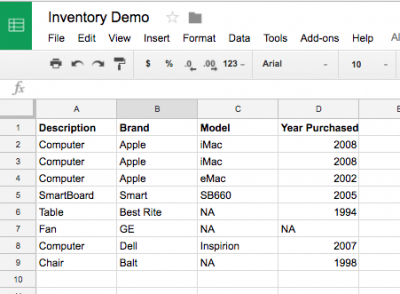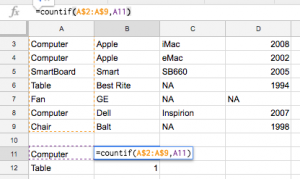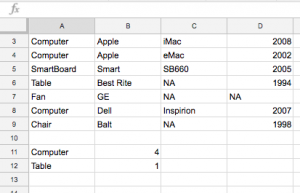### Google Sheets: Counting Occurrences of a String

Posted on October 26, 2016

Lot’s of us use spreadsheets to make lists. Sometimes it’s helpful to be able to count how many times something is in your list. Let’s say you are doing an inventory where you record:

• Description
• Brand
• Year Purchased

Maybe you have a spreadsheet like this:If you wanted to count how many times “Computer” was in your list, you can add the following below your list (in this example I’m putting the entry in cell A11). Just put the label of what string you want to count, then in the next column use the countif function like this: =countif(A2:A9,A11)

Let me explain a couple of things. First, the function is “countif” which means it will only count how many times in the range A2 to A11 that the string in cell A11 occurs. In other words, the count is 4 since “Computer” (which is in cell A11) in found in cells A2, A3, A4 and A8. Then you have this:By adding “\$” characters in front of the row numbers, you can indicate that the cell reference is “fixed” instead of “relative” and that allows you to add other strings from your list below the “Computer” entry and then just copy the formula in cell B11 downward.Click on the square in the bottom-right corner of cell B11 and drag downward to copy the formula to count other words:Now, if this isn’t fun then tell me what is.